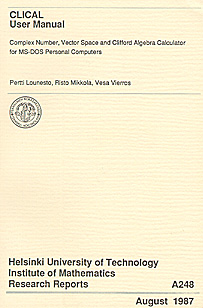CLICAL, a calculator type computer program for vectors, complex numbers, quaternions, bivectors, spinors, and multivectors in Clifford algebras

#### Department of Mathematics, University of Helsinki

CLICAL is a stand-alone calculator-type computer program for geometric algebras of multivectors, called Clifford algebras. CLICAL evaluates elementary functions with arguments in complex numbers, and their generalizations: quaternions, octonions and multivectors in Clifford algebras. CLICAL works directly on intrinsic geometric objects: lines, planes and volumes, represented by vectors, bivectors and multivectors. Oriented volume elementes, or segments of subspaces, are represented by simple multivectors, which are homogeneous and decomposable elements in the exterior algebra. CLICAL works on Clifford algebras Clp,q of real non-degenerate quadratic spaces Rp,q.

Clifford algebras are used to handle rotations and oriented subspaces. Clifford algebra is a user interface, which provides geometrical insight. However, the actual numerical computations are faster in matrix images of Clifford algebras. CLICAL computer program was developed to enable input-output in Clifford algebras (and fast internal computation in matrices).

CLICAL is intended for researchers and teachers of Clifford algebras and spinors. In research, CLICAL has been applied to verify and falsify conjectures about Clifford algebras. With the help of CLICAL, I have found counterexamples to conjectures and theorems about Clifford algebras. I have used CLICAL to solve problems presented in Usenet newsgroups, for instance about rotations of the 4D Euclidean space R4. In teaching, CLICAL has been used in mathematics and physics courses in the USA, Mexico, Finland and Spain. Take a look at a course delivered with CLICAL. There are competing projects, most notably an online geometric calculator, two symbolic computer algebra packages for MapleV5, one for Mathematica, MatLab geometric algebra tutotial, and C++ Template Classes for Geometric Algebras.

Download a zip-file (120kB) of CLICAL. In Windows Explore, click the file CLICAL (1kB), not CLICAL1 (120kB). In MS-DOS, type

C:\> clical

> help
> tutor
> get guide

In Macintosh, you need Windows and MS-DOS emulators, and possible upgrading of your Mac-OS. In UNIX, you need to support MS-DOS under X-Windows. The I/O of CLICAL is written in PASCAL. The numerical routines rely on Netlib's FORTRAN-package EISPACK.

Examples: 1. Find the distance of the point P = (2,3,1) from the line AB, where A = (1,2,0), B = (3,0,-2).

> dim 3
> P = 2e1+3e2+e3
> A = e1+2e2
> B = 3e1-2e3
> abs(((P-B)^(A-B))/(A-B))
ans = 1.633
[= sqrt(8/3)]

2. Compute i/(j+exp(kp/6)) in quaternions. Hint: Go to the Clifford algebra Cl0,3 and use the correspondences i = e1, j = e2, k = e3.

> dim 0,3
> q(u) = Re((1-e123)u)+Pu(1,(1-e123)u)
> q(e1/(e2+exp(pi/6 e3)))
ans = 0.433e1+0.250e2-0.5e3
[= sqrt(3)/4 i + 1/4 j - 1/2 k]

3. Find matrices of the two isoclinic rotations (= turns each plane the same angle) UL(a) = b, UR(a) = b sending a = (16+12i+5j+4k)/21 to b = (18+10i+4j+k)/21. Compute ba-1 = (1/441)(432-67e1+2e2-58e3), a-1b = (1/49)(48-5e1-6e2-6e3) and the components of (ba-1)q, q(a-1b) for q = 1, e1, e2, e3 to find the entries of

 UL = (1/441) [432 -67 2 -58 67 432 -58 -2 -2 58 432 -67 58 2 67 432],
 UR = (1/49) [48 -5 -6 -6 5 48 6 -6 6 -6 48 5 6 6 -5 48].
4. Find the matrix of the simple rotation (= turns only one plane) of R4 sending a = (16/21,12/21,5/21,4/21) to b = (18/21,10/21,4/21,1/21).

> dim 4
> a = (16e1+12e2+5e3+4e4)/21
> b = (18e1+10e2+4e3+e4)/21
> s = sqrt(b/a)
s = 0.995+0.064e12+0.030e13+0.064e14+0.002e23+0.032e24+0.013e34

[= (873+56e12+26e13+56e14+2e23+28e24+11e34)/sqrt(769986)]

Then compute Sij = ei.(sej/s) to find the matrix of the simple rotation:

 S = (1/42777) [42005 -5612 -2578 -5226 5252 42341 -390 -3062 2466 -2 42688 -1235 5638 2370 899 42328].
5. Form the matrix of the rotation, which is a composition of the four hyperplane reflections along e4-e3, e3-e2, e2-e1, e1.

> a = e1(e2-e1)(e3-e2)(e4-e3)
a = 1-e12+e13-e14-e23+e24-e34+e1234
> a e1/a
ans = e2
> a e2/a
ans = e3
> a e3/a
ans = e4
> a e4/a
ans = -e1

Thus, the rotation matrix is [0 0 0 -1,1 0 0 0,0 1 0 0,0 0 1 0]. The rotation turns one plane by angle 3p/4 and its orthogonal complement by angle p/4, the latter plane being e12+sqrt(2)e13+e14+e23+sqrt(2)e24+e34. The rotation sends the plane e12 to e23, e23 to e34, e34 to e14 and e14 to e12.

6. A 2-plane P in R4 is called a T-plane of a rotation R, if R(P)UP is a line, the line being called a T-line and the rotation being called a T-rotation. Are all non-isoclinic rotations T-rotations? Are all lines not in the invariant planes T-lines? Are all non-invariant planes T-planes? No (simple rotations of angle p are not), yes (for simple rotations of angle p and isoclinic rotations all lines are in invariant planes) and no. Take any line L outside of the two rotation planes of a non-isoclinic rotation R and consider the plane R-1(L)^L. Then R(R-1(L)^L) = L^R(L). Through a T-line there are exactly two T-planes.

#### Bibliography

P. Lounesto: Clifford Algebras and Spinors. Cambridge University Press, Cambridge, 1997, 1998, (2nd ed.) 2001.

P. Lounesto, R. Mikkola, V. Vierros: CLICAL User Manual: Complex Number, Vector Space and Clifford Algebra Calculator for MS-DOS Personal Computers. Institute of Mathematics, Helsinki University of Technology, 1987. 80 pages.

Student Guide of CLICAL, page 1, 2-3, 4-5, 6-7, 8-9, 10-11, 12-13, 14-15, 16-17, 18-19, 20-21, 22-23, 24-25, 26-27. Changes between versions 2/4.

March 1997 (last revised May 2002)